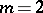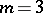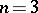# Form

(diff) ← Older revision | Latest revision (diff) | Newer revision → (diff)

A polynomial in several variables all terms of which are of the same degree.

Depending on the numberof variables, forms are called binary (for), ternary (for), etc.; depending on the degreeof their terms, they are called linear (for), quadratic (for), cubic (for), etc. If the variables can be split into groups so that each term of the form depends linearly on the variables in each group, then the form is called a multilinear form. Every form can be obtained from a multilinear form by identifying certain variables. Conversely, from each form one can obtain a multilinear form by a certain process, known as the polarization process.

The most important forms for applications are quadratic forms (cf. Quadratic form). The theory of quadratic forms is closely connected with that of second-order curves and surfaces (see also Hermitian form).

In number theory the question of whether integers can be represented as values of forms with integer coefficients for integer values of the variables is highly important, Fermat's theorem (cf. Fermat great theorem) is an example.

In differential and Riemannian geometry one uses differential forms (cf. Differential form). Many theorems of integral calculus (see Green formulas; Ostrogradski formula; Stokes formula) can be regarded as theorems on the connections between differential forms of different degrees.

How to Cite This Entry:
Form. Encyclopedia of Mathematics. URL: http://encyclopediaofmath.org/index.php?title=Form&oldid=14040
This article was adapted from an original article by Material from the article "Form" in BSE-3 (originator), which appeared in Encyclopedia of Mathematics - ISBN 1402006098. See original article